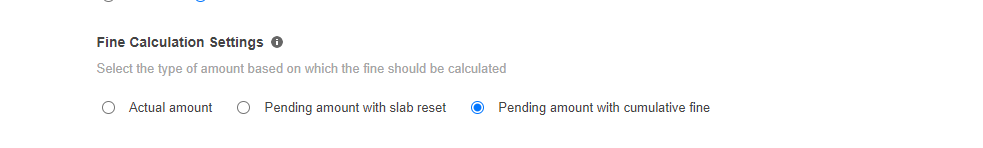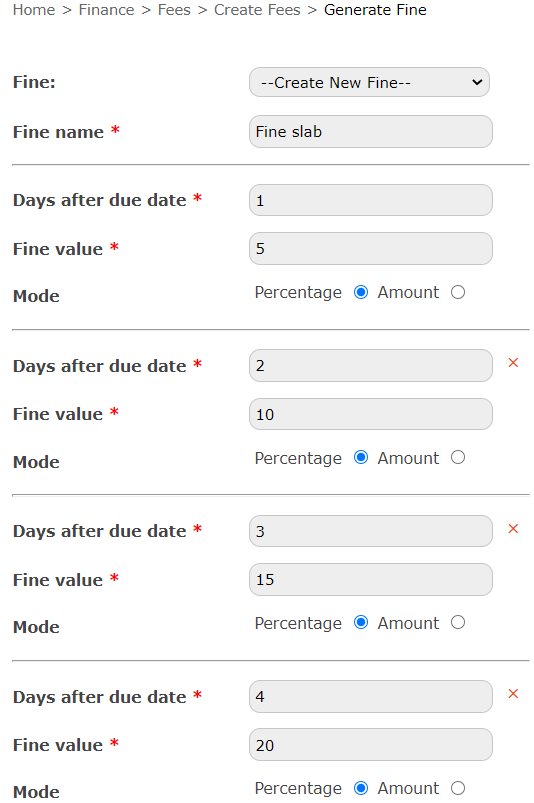# Cumulative Fine Calculation

Modified on Thu, 13 Jan 2022 at 02:07 PM

With this new feature, the fine will be calculated based on the pending amount, the fine calculated on each day after the due date will be stored separately. Fine will be deducted only after the principal amount is paid completely.

NOTE: To use this the "Pending Amount with Cumulative Fine" option should be enabled in Finance Settings.

Let's see how to manage Cumulative Fine Calculation in Fedena:

Home>>Finance>>Finance Settings>>General SettingsHere the fine for each day will be calculated based on the principal amount/pending amount, that is, excluding the fine. Also, when a student pays an amount, it will be deducted from the principal amount only. The calculated fine on each day will be added to the existing fine, this value will be kept separate throughout until the principal amount is paid. The fine calculation will stop once the principal amount/pending amount is 0.

Note: The fee collections will follow the settings that was enabled at the time of fee creation.

1. The amount paid by the student will be deducted from the pending amount(excluding fine)

2. Fine will be calculated based on the pending principal amount(excluding existing calculated fine) and will be shown in a separate column/row on the payment page(cumulative fine)

3. After the principal amount is paid off, a fine payment will be considered.Indicating the feature with an example

Considering the below fine slab and the principle amount as 800.Day 1;

fine=5%(800)=40/-

pending amount= 800/-

No Payment made by the student.

Day 2;

fine=10%(800)+40= 80+40= 120/-

Pending amount= 800/-

No Payment

Day 3;

If the student decides to pay some amount

Fine= 15%(800)+120= 120+120= 240/-

Paid= 200/-

Pending amount=800-200= 600/-

Day 4;

If the student decides to pay some amount

Fine= 20%(600)+240= 120+240= 360/-,

Paid= 200/-,

Pending amount=600-200= 400/-,

Day 5;

Fine= 25%(400)+ 360= 100+360= 460/- ,

Paid= 200/-,

Pending amount=400-200= 200/-,

Day 6;

No payment,

Fine= 30%(200)+460= 60+460= 520/-,

Pending amount= 200/-

Day 7;

If the student decides to pay

Fine= 35%(200)+520= 70+520= 590/-

Paid= 200/-

Pending amount=200-200= 0

Day 7;

As the student has paid the pending amount/principal amount completely, the fine calculation will stop.

Pending amount=0

Total fine=590/-

Amount to be paid= 590/-.